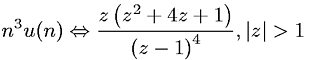Equations > Signal Processing > Z Transform Pairs > Z-transform involving the unit step function

### Z-transform involving the unit step functionLatex Code:

MathML Code:

 ${n}^{3}u\left(n\right)⇔\fracz\left({z}^{2}+4z+1\right){\left(z-1\right)}^{4},|z|>1$

MathType 5.0: